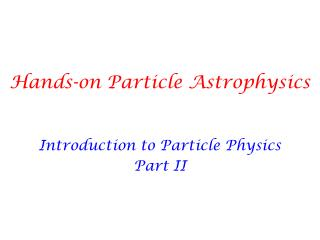DownloadDownload PresentationHands-on Particle Astrophysics

# Hands-on Particle Astrophysics

Télécharger la présentation## Hands-on Particle Astrophysics

- - - - - - - - - - - - - - - - - - - - - - - - - - - E N D - - - - - - - - - - - - - - - - - - - - - - - - - - -
##### Presentation Transcript

1. Hands-on Particle Astrophysics Introduction to Particle Physics Part II

2. Fermions (spin ½) Bosons (spin 1) Makeup hadrons baryons: mesons: electromagnetic strong weak

3. Smallest Building Blocks (Fermion Interactions) • Neutrinos (neutral leptons): • Charged leptons: • Quarks:

4. Smallest Building Blocks (Fermion Interactions) • Neutrinos (neutral leptons): Symmetries and conservation laws determine what is allowed • Charged leptons: • Quarks:

5. Smallest Building Blocks (Force Carrier Interactions) Electroweak interactions Z W W W W W W Z Z W W W W W W W Z W Gluon self-interactions g g g g g g g

6. Smallest Building Blocks (Force Carrier Interactions) Electroweak interactions Z W W W W W W Z Z W W W W W W W Z W Gluon self-interactions g g g Symmetries and conservation laws determine what is allowed g g g g

7. Symmetries of Standard Model • Excellent description of all interactions • Masses are not allowed! • Works well for photons/gluons • BUT W, Z massive • Fermions have mass! • Way out?

8. Symmetries of Standard Model • Excellent description of all interactions • Masses are not allowed! • Works well for photons/gluons • BUT W, Z massive • Fermions have mass! • Way out? “Hide” the symmetry • Interactions with new particle obey symmetries • the “ground state” is not symmetric • Familiar example: • gravitational interactions: spherical symmetry • (force: inverse square law) • and yet solar system not spherically symmetric

9. Simplest model – the standard model Higgs mechanism • specific allowed interactions • allows massive W’s, Z’s • allows fermion masses • specific relations among • - masses of W’s, Z • - interaction rates of various processes • + verified by data over last 40 years! • one spin 0 Higgs boson • specific • - production modes/rates • - decay modes/rates • unknown mass • discovered this year! • m = 125 GeV

10. H Smallest Building Blocks (Higgs Interactions) Higgs – fermion interactions H f f Higgs- force carrier interactions H H H H H H W W W Z Z W Z Z Higgs self-interactions H H H H H H H

11. LHC detectors ATLAS CMS